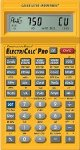Calculating Amps When Power Is In Watts

A common problem faced by many homeowners when doing renovations that involve the addition of electrical circuits is calculating the size of the circuit necessary to carry enough power to the electrical devices being used.

Many home appliances and other electrical devices rate their power requirement or dissipation in watts. A watt is a measure of energy, its electrical symbol is a capital.

The power information is usually located on an information plate on the device, however, there are exceptions. Items such as light bulbs do not provide power requirements on the device itself, it is left to the homeowner or electrician to calculate the current required.

With appliances and other electrical devices, defining their power requirements in watts and an electrical panel requiring fuses or breakers that are defined in amps, the process of determining the size of the fuse or breaker necessary to power a device or number of devices can be confusing.

It should be noted that many major appliance manuals will state a fuse size, and this should be followed.

As an example: You are installing a number of recessed light fixtures in your finished basement renovation and need to determine how many fixtures you can power from a 15 amp circuit.

The formula is: W ÷ E = IFigure 1 - Electrical calculator

60 (bulb wattage) divided by 120 (voltage) equals 1/2 amps. Hence, a 15 amp circuit could power 30 - 60 watt light bulbs.

If the light bulbs you were planning on using were only 15 watts the current required for each bulb would be 15 (bulb wattage) divided by 120 (voltage) equals 1/8 amps. Hence, a 15 amp circuit could power 120 - 15 watt light bulbs.

Of course a circuit should never be installed based on a 100% initial load. 50% or less is much more realistic. So, for 60 watt light bulbs, the maximum on a circuit should be 15 and for 15 watt - 60 light bulbs.

If you are working on a large remodel with a lot of electrical calculations, you may wish to consider the purchase of a calculator designed specifically for electrical calculations, as shown in Figure 1.

The formula works the same for any appliance or electrical device including furnaces and hot-water tanks.